Next: 4.1.1 Calculation of Conductances Up: 4. Simulator Coupling Previous: 4. Simulator Coupling

# 4.1 The Two-Level Newton Algorithm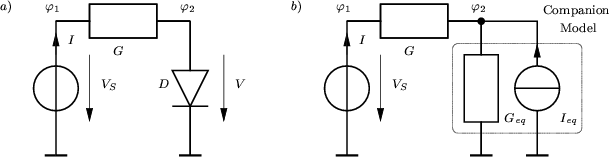The problem of a two-level device and circuit simulation is demonstrated by an example circuit as shown in Fig. 4.1 where VS is a constant voltage source and G is a linear conductance. The non-linear device is a diode whose characteristic is determined by the doping profile and the physical models used in the device simulation. The DC characteristic is shown in Fig. 4.2. In Fig. 4.1a the original circuit is shown. When using Newton's method to solve the non-linear circuit equations, a linear companion circuit  is solved at each iteration until some convergence criterion is met. The companion circuit for arbitrary non-linear two-terminal devices is shown in Fig. 4.1b. It consists of a linear conductance Geq and a current source Ieq which depend on the operating point. They are derived from the non-linear characteristic as shown in Fig. 4.2 and read

 Gk + 1eq =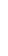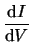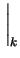(4.1) Ik + 1eq = Ik - Gk + 1eq . Vk (4.2)

with k being the iteration number of the Newton algorithm. For compact models, Geq and Ieq are evaluated analytically. As this is not possible for distributed devices, the device simulator has to be called for each Newton iteration. The node voltages are initialized to values supplied by the user and default to zero. After this initialization, the device simulator has to be called to calculate the companion model.

For the calculation of the conductances an approach as proposed in  is well suited and will be outlined in the following section.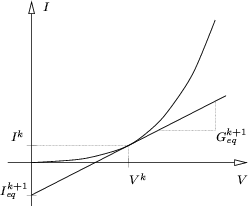Next: 4.1.1 Calculation of Conductances Up: 4. Simulator Coupling Previous: 4. Simulator Coupling
Tibor Grasser
1999-05-31